Updating search results...

# 60 Results

View
Selected filters:
• SlopeUnrestricted Use
CC BY
Rating
0.0 stars

This contemporary calculus course is the third in a three-part sequence. In this course students continue to explore the concepts, applications, and techniques of Calculus - the mathematics of change. Calculus has wide-spread application in science, economics and engineering, and is a foundation college course for further work in these areas. This is a required class for most science and mathematics majors.Login: guest_oclPassword: ocl

Subject:
Calculus
Material Type:
Full Course
Homework/Assignment
Lecture Notes
Lesson Plan
Syllabus
Provider:
Washington State Board for Community & Technical Colleges
Provider Set:
Open Course Library
10/31/2011Educational Use
Rating
0.0 stars

Students are introduced to the "Walk the Line" challenge question. They write journal responses to the question and brainstorm what information they need to answer the question. Ideas are shared with the class (or in pairs and then to the class, if class size is large). Then students read an interview with an engineer to gain a professional perspective on linear data sets and best-fit lines. Students brainstorm for additional ideas and add them to the list. With the teacher's guidance, students organize the ideas into logical categories of needed knowledge.

Subject:
Engineering
Mathematics
Material Type:
Lesson Plan
Provider:
TeachEngineering
Provider Set:
TeachEngineering
Author:
Aubrey Mckelvey
09/18/2014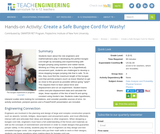Educational Use
Rating
0.0 stars

Students learn about the role engineers and mathematicians play in developing the perfect bungee cord length by simulating and experimenting with bungee jumping using washers and rubber bands. Working as if they are engineers for a (hypothetical) amusement park, students are challenged to develop a show-stopping bungee jumping ride that is safe. To do this, they must find the maximum length of the bungee cord that permits jumpers (such as brave Washy!) to get as close to the ground as possible without going "splat"! This requires them to learn about force and displacement and run an experiment. Student teams collect and plot displacement data and calculate the slope, linear equation of the line of best fit and spring constant using Hooke's law. Students make hypotheses, interpret scatter plots looking for correlations, and consider possible sources of error. An activity worksheet, pre/post quizzes and a PowerPoint® presentation are included.

Subject:
Mathematics
Statistics and Probability
Physical Science
Physics
Material Type:
Activity/Lab
Provider:
TeachEngineering
Provider Set:
TeachEngineering
Author:
Marc Frank
02/17/2017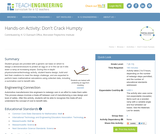Educational Use
Rating
0.0 stars

Student groups are provided with a generic car base on which to design a device/enclosure to protect an egg on or in the car as it rolls down a ramp at increasing slopes. During this in-depth physics/science/technology activity, student teams design, build and test their creations to meet the design challenge, and are expected to perform basic mathematical calculations using collected data, including a summative cost to benefit ratio.

Subject:
Engineering
Physics
Material Type:
Activity/Lab
Provider:
TeachEngineering
Provider Set:
TeachEngineering
Author:
Justin Riley
Ryan St. Gelais
Scott Beaurivage
09/18/2014Conditional Remix & Share Permitted
CC BY-NC-SA
Rating
2.0 stars

Students, in partners, will use an online graphing program to complete this discovery lesson. Each pair will change one component of an equation at at time and then complete a worksheet to describe the effect of the change.

Subject:
Mathematics
Material Type:
Activity/Lab
Author:
Donna Parrish
06/20/2016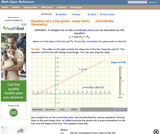Rating
0.0 stars

An interactive applet and associated web page that demonstrate the equation of a line in point-slope form. The user can move a slider that controls the slope, and can drag the point that defines the line. The graph changes accordingly and equation for the line is continuously recalculated with every slider and / or point move. The grid, axis pointers and coordinates can be turned on and off. The equation display can be turned off to permit class exercises and then turned back on the verify the answers. The applet can be printed as it appears on the screen to make handouts. The web page has a full description of the concept of the equation of a line in point - slope form, a worked example and has links to other pages relating to coordinate geometry. Applet can be enlarged to full screen size for use with a classroom projector. This resource is a component of the Math Open Reference Interactive Geometry textbook project at http://www.mathopenref.com.

Subject:
Geometry
Material Type:
Simulation
Provider:
Math Open Reference
Author:
John Page
02/16/2011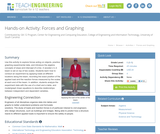Educational Use
Rating
0.0 stars

Use this activity to explore forces acting on objects, practice graphing experimental data, and introduce the algebra concepts of slope and intercept of a line. A wooden 2 x 4 beam is set on top of two scales. Students learn how to conduct an experiment by applying loads at different locations along the beam, recording the exact position of the applied load and the reaction forces measured by the scales at each end of the beam. In addition, students analyze the experiment data with the use of a chart and a table, and model/graph linear equations to describe relationships between independent and dependent variables.

Subject:
Engineering
Mathematics
Physics
Material Type:
Activity/Lab
Provider:
TeachEngineering
Provider Set:
TeachEngineering
Author:
Ivanka Todorova
Jed Lyons
09/18/2014
Remix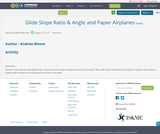Conditional Remix & Share Permitted
CC BY-NC-SA
Rating
0.0 stars

Students will calculate the glide slope ratio and angle of paper airplanes they have created. They will make claims about the type of airplane and support claims with evidence and reasoning from their class data.

Subject:
Algebra
Material Type:
Activity/Lab
Author:
04/29/2021Unrestricted Use
CC BY
Rating
0.0 stars

Student-facing 8th grade math resources. Covers transformations & congruence, slope, similarity, linear relationships, associations in data, volume, functions, scientific notation, the pythagorean theorem and irrational numbers.

Subject:
Mathematics
Material Type:
Unit of Study
Provider:
Open Up Resources
05/21/2019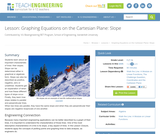Educational Use
Rating
0.0 stars

Students learn about an important characteristic of lines: their slopes. Slope can be determined either in graphical or algebraic form. Slope can also be described as positive, negative, zero or undefined. Students get an explanation of when and how these different types of slope occur. Finally, they learn how slope relates to parallel and perpendicular lines. When two lines are parallel, they have the same slope and when they are perpendicular their slopes are negative reciprocals of one another.

Subject:
Engineering
Mathematics
Algebra
Material Type:
Lesson Plan
Provider:
TeachEngineering
Provider Set:
TeachEngineering
Author:
Aubrey Mckelvey
09/18/2014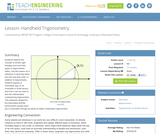Educational Use
Rating
0.0 stars

Students explore the concept of similar right triangles and how they apply to trigonometric ratios. Use this lesson as a refresher of what trig ratios are and how they work. In addition to trigonometry, students explore a clinometer app on an Android® or iOS® device and how it can be used to test the mathematics underpinning trigonometry. This prepares student for the associated activity, during which groups each put a clinometer through its paces to better understand trigonometry.

Subject:
Engineering
Mathematics
Trigonometry
Material Type:
Lesson Plan
Provider:
TeachEngineering
Provider Set:
TeachEngineering
Author:
Scott Burns
09/18/2014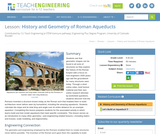Educational Use
Rating
0.0 stars

Students see that geometric shapes can be found in all sorts of structures as they explore the history of the Roman Empire with a focus on how engineers 2000 years ago laid the groundwork for many structures seen today. Through a short online video, brief lecture material and their own online research directed by worksheet questions, students discover how the Romans invented a structure known today as the Roman arch that enabled them to build architecture never before seen by humankind, including the amazing aqueducts. Students calculate the slope and its total drop and angle over its entire distance for an example aqueduct. Completing this lesson prepares students for the associated activity in which teams build and test model aqueducts that meet specific constraints. This lesson serves as an introduction to many other geometry—and engineering-related lessons—including statics and trusses, scale modeling, and trigonometry.

Subject:
Mathematics
Geometry
Material Type:
Lesson
Provider:
TeachEngineering
Author:
Lauchlin Blue
Malinda Zarske
Nathan Coyle
02/07/2017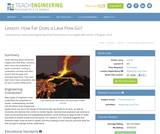Educational Use
Rating
0.0 stars

While learning about volcanoes, magma and lava flows, students learn about the properties of liquid movement, coming to understand viscosity and other factors that increase and decrease liquid flow. They also learn about lava composition and its risk to human settlements.

Subject:
Engineering
Mathematics
Physics
Material Type:
Lesson Plan
Provider:
TeachEngineering
Provider Set:
TeachEngineering
Author:
Brittany Enzmann
09/18/2014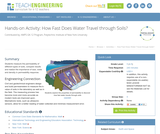Educational Use
Rating
0.0 stars

Students measure the permeability of different types of soils, compare results and realize the importance of size, voids and density in permeability response.

Subject:
Engineering
Mathematics
Material Type:
Activity/Lab
Provider:
TeachEngineering
Provider Set:
TeachEngineering
Author:
Eduardo Suescun
Magued Iskander
Russ Holstein
Ryan Cain
09/18/2014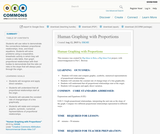Conditional Remix & Share Permitted
CC BY-NC-SA
Rating
0.0 stars

Students will use ratios to demonstrate the connections between proportional relationships, lines, and linear equations. Students will solve problems using a cooperative, kinesthetic activity in which they will create a ratio table, then graph proportional relationships with their bodies to demonstrate that the ratio (or rate) is the slope that will always pass through the origin.

Subject:
Mathematics
Material Type:
Activity/Lab
Teaching/Learning Strategy
Author:
01/28/2016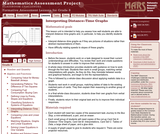Only Sharing Permitted
CC BY-NC-ND
Rating
4.0 stars

This lesson unit is intended to help teachers assess how well students are able to interpret distanceĐtime graphs and, in particular, to help you identify students who: interpret distanceĐtime graphs as if they are pictures of situations rather than abstract representations of them; and have difficulty relating speeds to slopes of these graphs.

Subject:
Mathematics
Material Type:
Assessment
Lesson Plan
Provider:
Shell Center for Mathematical Education
Provider Set:
Mathematics Assessment Project (MAP)
04/26/2013
RemixConditional Remix & Share Permitted
CC BY-NC-SA
Rating
0.0 stars

Throughout this lesson, the teacher will explain equivalent fractions, plot them on a coordinate plane, connect and extend those points, then discuss the importance of the relationship and explore what the students notice about the relationship. This lesson can help depend understanding of equivalent fractions as well as deepen the discussion into slope being similar to the fraction that we gave at the beginning of the lesson. (Math Solutions Training idea)

Subject:
Mathematics
Material Type:
Activity/Lab
Author:
Cortney Ray
08/08/2019Conditional Remix & Share Permitted
CC BY-NC-SA
Rating
0.0 stars

Throughout this lesson, the teacher will explain equivalent fractions, plot them on a coordinate plane, connect and extend those points, then discuss the importance of the relationship and explore what the students notice about the relationship. (Math Solutions Training idea)

Subject:
Mathematics
Material Type:
Activity/Lab
Author:
Cortney Ray
01/28/2016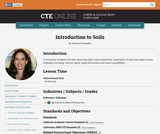Conditional Remix & Share Permitted
CC BY-NC-SA
Rating
0.0 stars

In this lesson students will learn about the basic soils components, importance of soils,main ideas in land evaluation including: texture, depth, slope and erosion and career possibilities.

Subject:
Agriculture
Material Type:
Lesson Plan
Provider:
Butte County Office of Education
Provider Set:
CTE Online
Author:
Jessica Fernandes
10/10/2016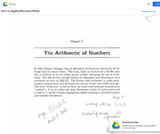Unrestricted Use
CC BY
Rating
0.0 stars

Introductory Algebra is the second of three classes in the developmental mathematics sequence. It provides a development of concepts of variables, expressions, and equations using symbolic algebra to represent primarily linear relationships both graphically and analytically. The concept of function will be developed for the application of linear equations and concepts of dependent and independent variable. Students will also learn to solve simultaneous linear equations as well as how to construct linear equations from slope and point information. Application problems will include geometric figure quantities, ratio and proportion, direct and indirect variation, and conversion of units. Finding the greatest common factor of a polynomial will also be included. The emphasis of the three semester sequence is fortification of mental calculation power with minimum reliance on digital calculation.

Subject:
Algebra
Material Type:
Full Course
Provider:
MassBay Community College
Author:
Ravindra Bhalla